﻿ Wave Perturbations for Linear Instability of Magneto Hydrodynamic Hartman Flow between Parallel Plates

# Journal of Physical MathematicsOpen Access

• Research Article
• J Phys Math 2019, Vol 10(3): 307

# Wave Perturbations for Linear Instability of Magneto Hydrodynamic Hartman Flow between Parallel Plates

Zakir Hussain1,2, Huisheng Zhang2 and Sergei Zuev3,4,5*
2Department of Biomedical Engineering, School of Medicine, Shenzhen University, Shenzhen, P.R. China
4Belgorod State Technological University, V.G. Shoukhov, Belgorod, Russia
5Crimean Federal University, Simferopol, Crimea
*Corresponding Author: Sergei Zuev, Belgorod State Technological University, V.G. Shoukhov, Belgorod, Russia, Tel: +7 477 2372742, Email: [email protected]

Received Date: Aug 28, 2019 / Accepted Date: Sep 28, 2019 / Published Date: Oct 15, 2019

### Abstract

The Hartmann layers of conducting fluid between parallel plates under the influence of the transvers magnetic field are considered. The standard boundary conditions with zero velocities on the plates are used. The perturbations are used to obtain the exact solution for the given system. It is shown explicitly that no instability near the stationary solution can be found by perturbations in 2-dim cases. In 3-dim case the instability arises just when quaternionic perturbations applied. We use analytical solutions to obtain explicit results and wave perturbations for considered system. Our results are agreed with experimental facts which was solved by other authors and confirms the wellknown fact that traversal magnetic field overcomes the instability. Similarly we obtained the exact solution by using new quaternionic method and this way of nonlinear system consideration can deliver a variety of possibilities for finding new solutions.

Keywords: MHD; Conductive fluid; Hartmann layer instability; Hartmann flow; Exact solution; Quaternionic continuation

#### Introduction

The Hartmann layer is a significant element of magnetohydrodynamics (MHD). It is defined as the flow of an electrically conducting fluid along any boundary in the constant magnetic field that is not tangential to the boundary. The shear stress in such flow was mainly studied . Models of Hartmann layers are used in many situations, for example Hartmann boundary layer models study the transmission of heat and mass transfer of the fluid. Similarly, the global electric circulation is afflicted and the flow can completely change its nature and anxiety, if a laminar Hartmann layer is destabilized as it was shown .

In general, an influence of magnetic field to a boundary layer leads to two results on the layer stability. The first one is that magnetic field accelerates perturbations through Joule dissipation. The second one is the impact on the laminar velocity profile and hence on the critical wave number value. In particular, in the field of crystal disturbance, a steady magnetic field is used to stabilize the flow i.e, it minimize the instability . There should be some condition on magnetic field to be used to achieve the stability of Hartmann layers. In this work, we study some conditions on magnetic field which can be used to bring more stabilizing effects on the Hartmann layers. The results proposed in this work show some direction for proof.

In quantitative evaluation, the stability of the Hartmann layer can help to impove the understanding of the global magnetic field damping source . In the fusion reactor scheme, a so-called liquid metal blanket surrounds the plasma and is subjected to a strong magnetic field. The natural convection, which develops due to the large heat flow, produces maximum speeds and therefore the stability of the Hartmann layer should be explored. Finally, the case of MHD two-dimensional disturbance theory is associated with the Hartmann layer case. It is usually assumed that the layer is laminar and therefore easily obtains a linear damping force acting on the two-dimensional disturbance. If, however, the Hartmann layer becomes unstable, this linear term should be changed by another model .

A study  has considered the three-dimensional magnetoconvection disturbance for Rayleigh number = 107 in liquid gallium for very large external normal magnetic fields in direct numerical approximation by using quasistatic approach. They found that the instabilty of the convection flow decreases for some critical Hartmann numbers. The article  investigates a model for the turbulent Hartmann layer based on Prandtl’s mixing length model without adding arbitrary parameters. The authors found an exact expression of the displacement thickness of the turbulent Hartman layer.

In the present article, as usual, an unstable eigenvalue is an eigenvalue in the complex upper half plane, related to an eigenmode of the linearised problem that grows exponentially as a function of time t. It is natural to expect that a flow will behave unstably if and only if there exists such a growing eigenmode, and over the years much has been learned about which flows possess such modes. Corresponding distinctions in flows depend on the geometry, the Reynolds number, and sometimes other parameters.

As for numerical simulations, a study  used collocation method for strong magnetic field similarly to the Orr Sommerfeld system. Linear temporal stability analysis was investigated  to find the time expansion of small 2-dimensional disturbance was applied to the basic flow. The magnetic field influence was used by to investigate the instability of electrically conducting fluid. QR and QZ methods are considered to solve the complete eigenvalue technique . It was found that the stabilizing effect of the slip on the MHD flow is strong, although the slip length is very small if compared to the thickness of the Hartmann layer.

A careful survey of available literature reveals that even numerical studies of the stability of the basic flow and the growth of the small disturbance of plane Hartmann flow under magnetic field are still exploreable. Exact solutions in this field are also in scanty number. The solution proposed in this paper is applicable to the instability of the Hartman flow of an electrically conducting fluid under the influence of normal magnetic field. We are going to determine the equations which define the disturbance growth using the plane waves perturbation approach. The results should be qualitatively agreed with the experimental results of some articles [10,11], where the stability of these nonlinear problems in high frequencies was explored.

The article is organized as follows. In Section 2 we develop the mathematical model of the considered physical system. The solution of the corresponding system of differential equations is made in Section 3. Section 4 presents some discussions and summarizes the results of the article.

#### Mathematical Problem Formulation

We consider the magnetohydrodynamic instability of an electrically conducting, incompressible, and viscous fluid of Hartman flow in the presence of transversal magnetic field. The fluid is placed between upper and lower parallel plates by distance 2L. The fluid is flowing in the y-direction whereas velocity gradient is along x-axis. The uniform magnetic field applied in x-direction as presented in Figure 1.

The problem can be modelled by Navier-Stokes equation with external magnetic field. We use the following notations: the vector field of velocities is v, the electric current density is the vector field j, p is scalar function of the pressure and φ is electric scalar potential.

The governing equations for the model can be written as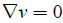(1)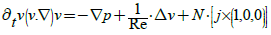(2)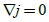(3)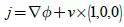(4)

The boundary conditions for the system are assumed as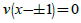(5)

and the electric potential φ satisfies Poisson’s eqns. (3) and (4) and satisfies following boundary conditions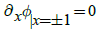(6)

The problem is described by two parameters: Reynolds number Re and interaction parameter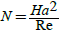, where Ha represents Hartmann number, determined by the definition of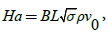Where σ, ρ and vo are electric conductivity, mass density and kinematic viscosity, respectively. As for magnetic magnitude B and size L, these values are equal to 1 in this work to make the simple speculations.

To start with the 2-dimensional problem: all functions treated as independent on z and perturbations are also depend on t,x,y only. It is shown further that there is no instability appears in this model with plane waves perturbations. Hence, to describe the observable phenomenas, the 3-dimensional case should be considered.

#### Solutions of the Governing Equations

Two dimensional case

Let us denote

v=(u,v,w)

When none function depends on z we have from (1-4) the following system of scalar equations: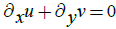(7)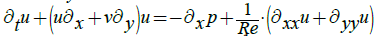(8)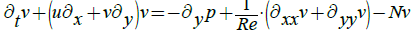(9)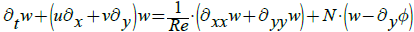(10)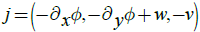(11)

with boundary conditions (5) and (6). In stationary case we can use Hartmann’s  solution which takes the following form with respect of the notations above: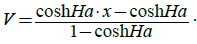(12)

The perturbed equation near the stationary Hartman solution are considered as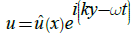(13)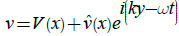(14)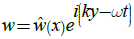(15)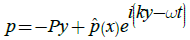(16)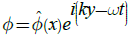(17)

Where V(x) is determined by (12) and xϵ[-1;1].

From (13-14) and (7) we have: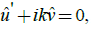(18)

where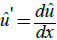. Use of (13), (14) and (16), eqn. (8) gives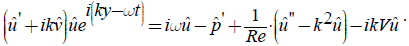which further gives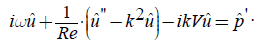Similarly, substituting (13), (14) and (16) in (9), we obtain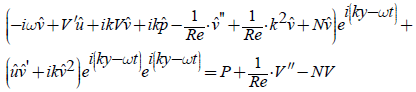Right side of latter equation is zero if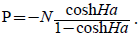Hence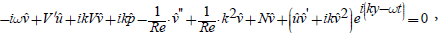Comparing y and t term, we get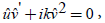we further get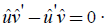(19)

Similarly, from (10), we have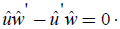(20)

Generally the functions uˆ, vˆ, wˆ can be obtained from (18-20) through integration, as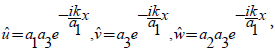(21)

Where a1,a2,a3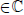are some constants. Note, that this result is obtained without using any condition, i.e., from wave perturbation eqns. (13)-(17) and MHD Navier-Stokes eqns. (7)-(11).

Combining (3), (11), (14), (15) and (17), we come to the following equation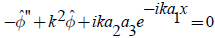with general solution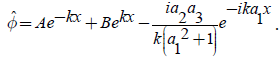This expression shows that no boundary conditions are necessary.

Now the eqn. (7) is identity and eqns. (8)-(10) turn to the next: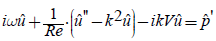(22)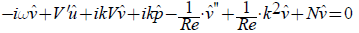(23)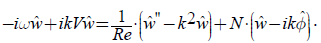(24)

Using relation (21) we have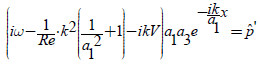(25)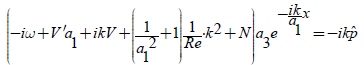(26)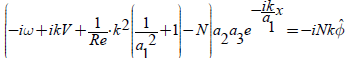(27)

Differentiating eqn. (26) and recalling that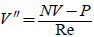, (25) gives the following relation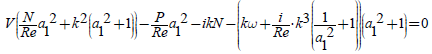From here, separating constants and variables, we have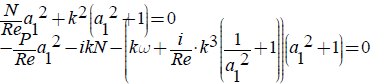Now we can calculate a1 and ω as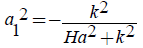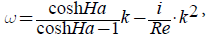(28)

and as a result, the imaginary part of the w is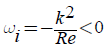(29)

This means that the flow is always stable.

Thus in case of plane waves perturbations, we have an exact solution (21), (26) and (27) of the system (7-11) without any turbulance. The turbulence can exist in the case of three dimension flow, by using the same technique. Let us come to 3-dimensional case.

Three dimensional case

To consider the non-restricted (by dimension) solution of the eqns. (1)-(6) it is natural to use the same perturbation idea but include wave with z coordinate: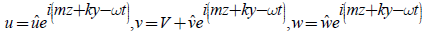, (30)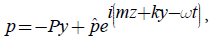(31)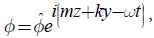(32)

all the functions depend on x with the same stationary solution Vdetermined by (12). The eqns. (1)-(5) now take the form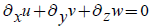(33)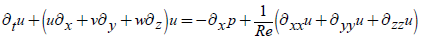(34)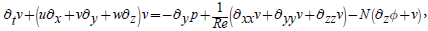(35)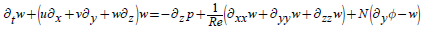(36)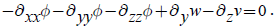(37)

After substitution of the eqns. (30)-(32) into the system (33-37) and all simplifications which are similar to those for 2-dimensional case, we correspondingly derive the following equations for the flow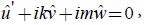(38)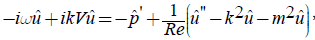(39)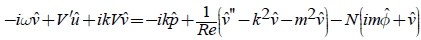, (40)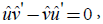(41)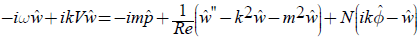, (42)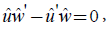(43)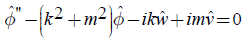(44)

And therefore for functions uˆ, vˆ, wˆ we have the next system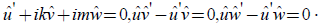(45)

It can be easily integrated and the general solution is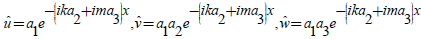(46)

Substituting these values in eqns. (39) and (42), we find that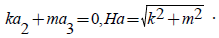(47)

Thus the solution for the system (38-39,41-44) is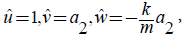(48)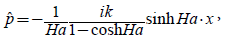(49)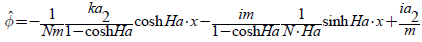, (50)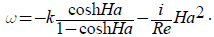(51)

The eqn. (40) is satisfied if Ha=0, but physically useless. The similar result can be obtained by solving the system (38-40,42-44), then we have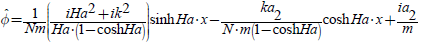Therefore, the classical plane wave perturbations leads to no solution and give no physical meanings. In the next section, we obtain analytical solution between critical wave number kc and and Hartmann number Ha. The litrature shows that MHD equation are solved only numerically and we have sovled the exact solution of Navier stokes equations by using quaternionic method.

Three dimensional case: quaternionic plane waves

For physical relevant solution in 3D case, we change the wave perturbation equations. To make the model more simple, we use complex representation instead of the trigonometric real functions. In the case of eqns. (1-6) the complex model does not give any solution because one of the equations does not satisfy. We can try to use the quaternionic plane waves instead of complex perturbations and therefore the new system takes the form: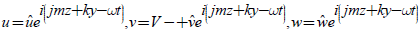, (53)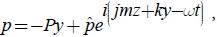(54)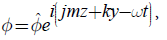(55)

Where, j is another one imaginary unit and ij=-ji and ω= ωr + jωj is some complex value with j imaginary unit. The physical values are real as well as in the complex case.

When the quaternionic plane waves perturbations (53-55) are replaced in system (1-6), we have the following equations instead of (38-44)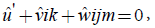(56)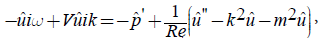(57)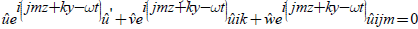, (58)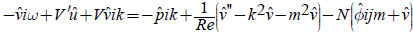, (59)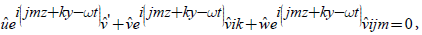(60)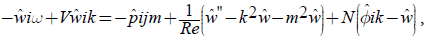(61)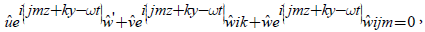(62)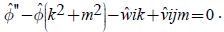(63)

Similar technique, as used in Section 3.2, can be applied to solve the latter system but it is necessary that the exponential factor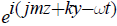is commutative with j imaginary unit. Then the solution becomes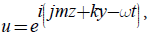(64)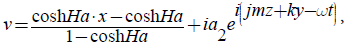(65)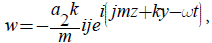(66)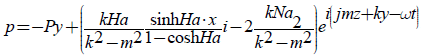, (67)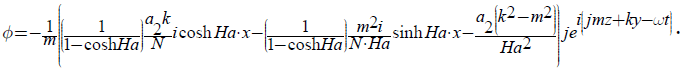(68)

These functions lead to solve identities all the eqns. (1-5) in quaternionic formulation. Also we have the following expressions for ω components: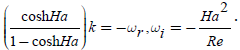The eqn. (6) can be obtained through special value of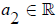. But in the expression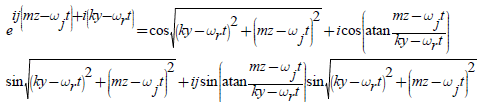, (70)

which is the Euler’s formula analogue, the real part should be zero. From above relation we have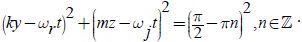(71)

Solving (71) for t, we find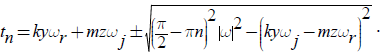(72)

Let us denote the fixed value of y,z and t by ξ,η,τ-respectively and we write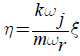Then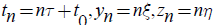and it is easy to show from (72) that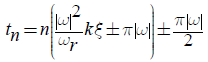i.e.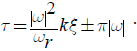(73)

When we turn back to the continious range of t, we should put τ = 0 Otherwise our solution functions are not continious. From (73) with τ = 0 and (69) we have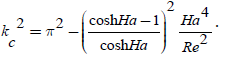(74)

Where we denote the critical wave number as kc.

#### Conclusion

In this work, we study Hartmann flow with a transverse strong magnetic field through wave perturbations. First, it is shown that in case of two dimensional flow perturbations do not provide unstable solution. In the second part we consider three dimentional flow in complex system and show that there is no solution for coresponding MHD equations. In the third section we obtain analytical solution between critical wave number kc and Hartmann number Ha. The litrature shows that MHD equation are solved only numerically and we have sovled the exact solution of Navier-Stokes equations by using quaternionic method.

The formula (74) gives the analitical dependance which is earlier was calculated numerically only. This formula is qualitatively correlates with the experimental observations and numerical simulations.

The approach which has used to obtain the solution can be used in other models with different kinds of equation systems. The quaternionic method also can be treated as the result of the article.

#### Acknowledgments

The research work was acknowledged by the Higher Education Commission Pakistan (PD-3006), the National Natural Science Foundation of China (11504238, 21706161), the Natural Science Foundation of Guangdong (2017A030310444), the Fundamental Research Program of Shenzhen (GRCK2017042110405436) and the Natural Science Foundation of SZU (grant no. 2017030).

#### References

Citation: Hussain Z, Zhang H, Zuev S (2019) Wave Perturbations for Linear Instability of Magneto Hydrodynamic Hartman Flow between Parallel Plates. J Phys Math 10: 306.

Copyright: © 2019 Hussain Z, et al. This is an open-access article distributed under the terms of the Creative Commons Attribution License, which permits unrestricted use, distribution, and reproduction in any medium, provided the original author and source are credited.

Select your language of interest to view the total content in your interested language

##### Recommended Journals
Viewmore
###### Article Usage
• Total views: 387
• [From(publication date): 0-0 - Dec 09, 2019]
• Breakdown by view type
• HTML page views: 360Can't read the image? click here to refresh